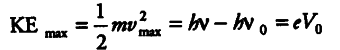# Write the important properties of photons

(i) Write the important properties of photons which are used to establish Einstein’s photoelectric equation.
(ii) Use this equation to explain the concept of (a) threshold frequency and (b) stopping potential.

(i) Important properties of photons which are used to establish Einstein’s photoelectric equations are as follows:
(a) In interaction of radiation with matter,radiation behaves as, if it is made up of particles called photons.
All photons of light of a particular frequency λ or wavelength A have the same energy
E=hv and momentum p = hv/c
whatever the intensity of radiation may be.
(ii) Since, Einstein’s photoelectric equation is given by(a) For a given material, there exist a certain
minimum frequency of the incident radiation, below which no emission of photoelectron takes place. This., frequency is called threshold frequency Above threshold frequency, the maximum kinetic energy of the emitted photoelectron or equivalent stopping potential is independent of the intensity of the incident light but depends only upon the frequency of the incident light.
(b) If the collecting plate in the photoelectric
apparatus is made at high negative potential, then most of the high energetic electrons get repelled back along the same path and the photoelectric current in the circuit becomes zero. So, for a particular frequency of incident radiation, the minimum negative potential for which the electric current becomes zero is called cut-off or stopping potential